# JEE Main 2021 Physics Paper With Solutions Feb 24 Shift 2

JEE Main 2021 Physics question paper for February 24, shift 2, is provided here to help students move in the right direction to prepare effectively and clear the entrance exam. Along with the question paper, students will find detailed solutions that have been meticulously prepared by our subject experts. Thus, students will get only accurate answers to each question and they will be able to learn as well as understand the discussed topic more clearly.
Students can view or download the JEE Main 2021 Physics question paper PDF from below. Alternatively, students can engage in solving the questions themselves before looking at the solutions. By doing this, they will not only get to know their preparation level, but they will develop greater speed and accuracy for solving different types of JEE Physics problems.

### JEE Main 2021 24th February Shift 2 Physics Question Paper

SECTION A

Question 1: Zener breakdown occurs in a p-n junction having p and n both:

1. a. lightly doped and have wide depletion layer.
2. b. heavily doped and have narrow depletion layer.
3. c. heavily doped and have wide depletion layer.
4. d. lightly doped and have narrow depletion layer.

Solution:

The zener breakdown occurs in the heavily doped p-n junction diode. Heavily doped p-n junction diodes have narrow depletion region. The narrow depletion layer width leads to a high electric field which causes the p-n junction breakdown.

Question 2: According to Bohr atom model, in which of the following transitions will the frequency be maximum?

1. a. n = 2 to n = 1
2. b. n = 4 to n = 3
3. c. n = 5 to n = 4
4. d. n = 3 to n = 2

Solution: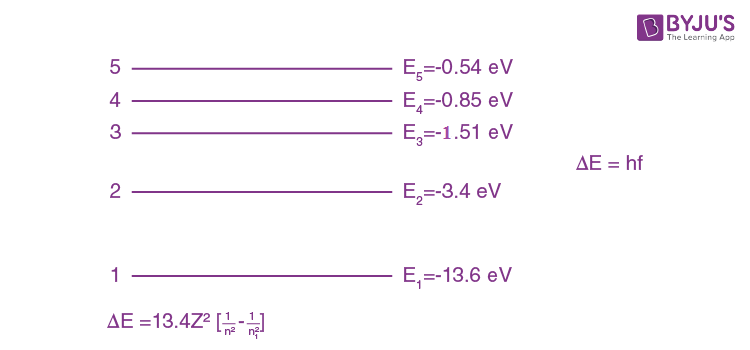Since, ΔE is maximum for the transition from n = 2 to n = 1

f is more for transition from n = 2 to n = 1.

Question 3: An X-ray tube is operated at 1.24 million volt. The shortest wavelength of the produced photon will be:

1. a. 10-2 nm
2. b. 10-3 nm
3. c. 10-4 nm
4. d. 10-1 nm

Solution:

The minimum wavelength of photon will correspond to the maximum energy due to accelerating by V volts in the tube.

λmin = hc/eV

λmin = 1240nm-eV/1.24×106

λmin = 10-3 nm

Question 4: On the basis of kinetic theory of gases, the gas exerts pressure because its molecules:

1. a. suffer change in momentum when impinge on the walls of container.
2. b. continuously stick to the walls of container.
3. c. continuously lose their energy till it reaches wall.
4. d. are attracted by the walls of container.

Solution:

Based on kinetic theory of gases, molecules suffer change in momentum when impinge on the walls of container. Due to this they exert a force resulting in exerting pressure on the walls of the container.

Question 5: A circular hole of radius (a/2) is cut out of a circular disc of radius ‘a’ shown in figure. The centroid of the remaining circular portion with respect to point ‘O’ will be: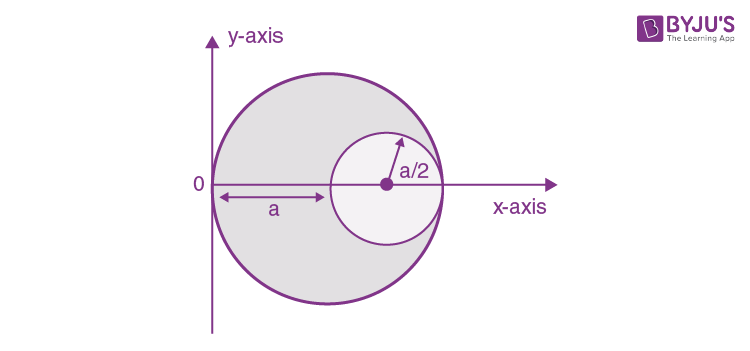1. a. (10/11)a
2. b. (⅔)a
3. c. (⅙)a
4. d. (⅚)a

Solution:

Let σ be the surface mass density of disc.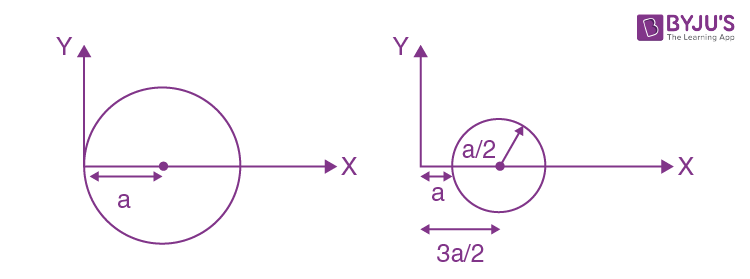Xcom= (m1x1 - m2x2)/(m1 - m2)

Where m = σπr2

Xcom= (σ×πa2×a) - (σπa2/4) × 3a/2)/(σπa2 - σπa2/4)

Xcom= (a - 3a/8)/(1 - ¼)

Xcom= (5a/8)/(¾)

Xcom= 5a/6

Question 6: Given below are two statements:

Statement I: PN junction diodes can be used to function as transistor, simply by connecting two diodes, back to back, which acts as the base terminal.

Statement II: In the study of transistor, the amplification factor β indicates ratio of the collector current to the base current.

In the light of the above statements, choose the correct answer from the options given below.

1. a. Statement I is false but Statement II is true.
2. b. Both Statement I and Statement II are true.
3. c. Statement I is true but Statement II is false.
4. d. Both Statement I and Statement II are false.

Solution:

S-1

Statement 1 is false because in case of two discrete back to back connected diodes, there are four doped regions instead of three and there is nothing that resembles a thin base region between an emitter and a collector.

S-2

Statement-2 is true, as

β = IC/IB

Question 7: When a particle executes SHM, the nature of graphical representation of velocity as a function of displacement is:

1. a. elliptical
2. b. parabolic
3. c. straight line
4. d. circular

Solution:

We know that in SHM;

V = ω√(A2 - x2)Elliptical

Alternate:

x = A sinωt ⇒ sin ωt = x/A

v = Aω cos ωt ⇒ cos ωt = v/Aω

Hence (x/A)2 + (v/Aω)2 = 1

Which is the equation of an ellipse.

Question 8: Match List - I with List - II.

 List – I List - II (a) Source of microwave frequency (i) Radioactive decay of nucleus (b) Source of infrared frequency (ii) Magnetron (c) Source of Gamma Rays (iii) Inner shell electrons (d) Source of X-rays (iv) Vibration of atoms and molecules - (v) LASER - (vi) RC circuit

Choose the correct answer from the options given below:

1. a. (a)-(ii),(b)-(iv),(c)-(i),(d)-(iii)
2. b. (a)-(vi),(b)-(iv),(c)-(i),(d)-(v)
3. c. (a)-(ii),(b)-(iv),(c)-(vi),(d)-(iii)
4. d. (a)-(vi),(b)-(v),(c)-(i),(d)-(iv)

Solution:

(a) Source of microwave frequency – (ii) Magnetron

(b) Source of infrared frequency – (iv) Vibration of atom and molecules

(c) Source of gamma ray – (i) Radioactive decay of nucleus

(d) Source of X-ray – (iii) inner shell electron

Question 9: The logic circuit shown below is equivalent to :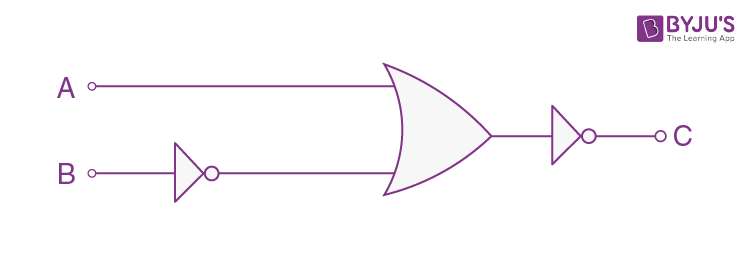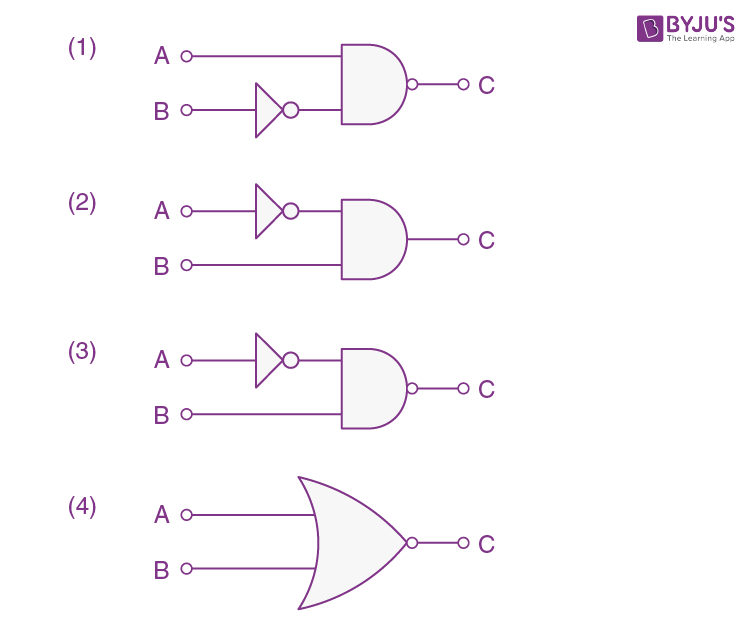Solution: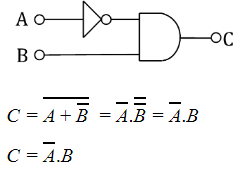Question 10: If the source of light used in a Young’s double slit experiment is changed from red to violet:

1. a. the fringes will become brighter.
2. b. consecutive fringe lines will come closer.
3. c. the central bright fringe will become a dark fringe.
4. d. the intensity of minima will increase.

Solution:

β = λD/d

As λvR

βv< βR

⇒ Consecutive fringe line will come closer.

⇒ (2)

Question 11: A body weighs 49 N on a spring balance at the north pole. What will be its weight recorded on the same weighing machine, if it is shifted to the equator?

[Use g = GM/R2 = 9.8 ms-2 and radius of earth, R = 6400 km.]

1. a. 49 N
2. b. 49.83 N
3. c. 49.17 N
4. d. 48.83 N

Solution:

At North Pole, weight

Mg = 49

Now, at equator

g’ = g - ω2R

⇒ Mg' = M(g - ω2R)

⇒ weight will be less than Mg at equator.

Alter:

g is maximum at the poles.

Hence from options only (d) has lesser value than 49N.

Question 12: If one mole of an ideal gas at (P1, V1) is allowed to expand reversibly and isothermally (A to B) its pressure is reduced to one-half of the original pressure (see figure). This is followed by a constant volume cooling till its pressure is reduced to one-fourth of the initial value (B→C). Then it is restored to its initial state by a reversible adiabatic compression (C to A). The net work done by the gas is equal to: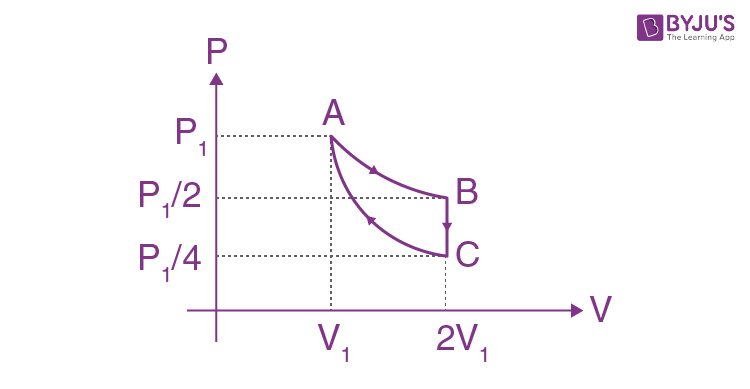1. a. 0
2. b. -RT/2( γ - 1)
3. c. RT [ln2 -1/2( γ -1)]
4. d. RT ln2

Solution:

AB → Isothermal process

WAB = nRT ln2 = RT ln2

BC → Isochoric process

WBC = 0

WCA = P1V1 - (P1/4)X2V1)/(1 - γ)

= P1V1/2(1 - γ)

= RT/2(1 - γ )

WABCA = RT ln2 + RT/2(1 - γ)

= RT [ln2 - 1/2(γ - 1)]

Question 13: The period of oscillation of a simple pendulum is T = 2π√(L/g). Measured value of ‘L’ is 1.0 m from meter-scale having a minimum division of 1 mm and time of one complete oscillation is 1.95 s measured from stopwatch of 0.01 s resolution. The percentage error in the determination of ‘g’ will be:

1. a. 1.33 %
2. b. 1.30 %
3. c. 1.13 %
4. d. 1.03 %

Solution:

T = 2π√(L/g)

T2 = 4𝜋2 [L/g]

g = 4𝜋2 [L/T2]

Δg/g = ΔL/L + 2ΔT/T

= [1mm/1m + 2(10×10-3)/1.95]×100

= 1.13 %

Question 14: In the given figure, a body of mass M is held between two massless springs, on a smooth inclined plane. The free ends of the springs are attached to firm supports. If each spring has spring constant k, the frequency of oscillation of given body is: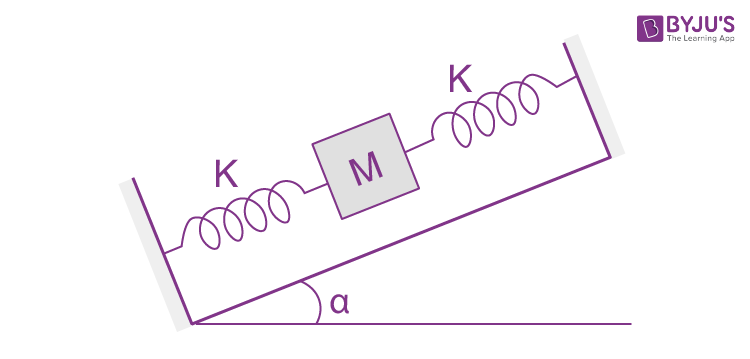1. a. (1/2π) √(2K/Mg sin α)
2. b. (1/2π) √(K/Mg sin α)
3. c. (1/2π) √(2K/M)
4. d. (1/2π) √(K/2M)

Solution:

Equivalent K = K + K = 2K

Now, T = 2π √(M/Keq)

⇒ T = 2π √(M/2K)

∴f = (1/2π) √(2K/M)

Question 15: Figure shows a circuit that contains four identical resistors with resistance R = 2.0 Ω. Two identical inductors with inductance L = 2.0 mH and an ideal battery with emf E = 9.V. The current ‘i’ just after the switch ‘s’ is closed will be: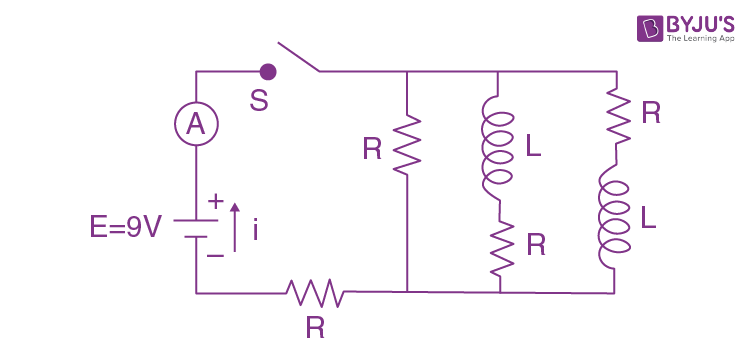1. a. 9A
2. b. 3.0 A
3. c. 2.25 A
4. d. 3.37 A

Solution:

Just when switch S is closed, inductor will behave like an infinite resistance. Hence, the circuit will be like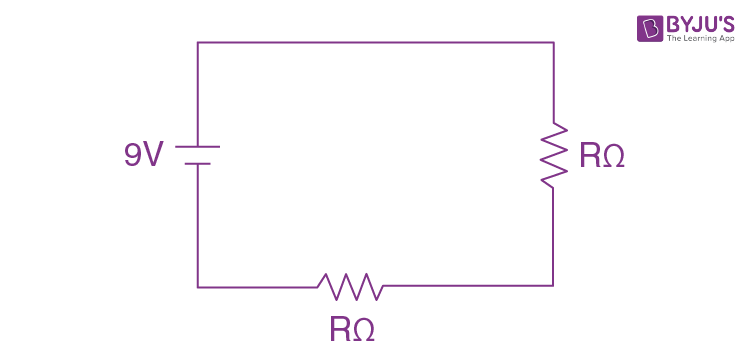Given: V = 9 V

From V = IR

I = V/R

Req. = 2 + 2 = 4 Ω

i = 9/4 = 2.25 A

Question 16: The de Broglie wavelength of a proton and α-particle are equal. The ratio of their velocities is:

1. a. 4:2
2. b. 4:1
3. c. 1:4
4. d. 4:3

Solution:

Sol.

From De-Broglie’s wavelength:-

λ = h/mv

Given λP = λα

v ∝ 1/m

vp/vα = mα/mp = 4mp/mp

= 4/1

Question 17: Two electrons each are fixed at a distance ‘2d’. A third charge proton placed at the midpoint is displaced slightly by a distance x (x<<d) perpendicular to the line joining the two fixed charges. Proton will execute simple harmonic motion having angular frequency:

(m = mass of charged particle)

1. a. (q2/2πε0md3)1/2
2. b. (πε0md3/2q2)1/2
3. c. (2πε0md3/q2)1/2
4. d. (2q2/πε0md3)1/2

Solution: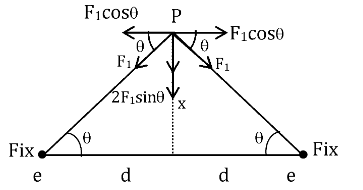Restoring force on proton:-

Fr = 2F1 sinθ where F1 = kq2/(d2 + x2)

Fr = 2Kq2x/[d2 + x2]3/2

x <<< d

Fr = 2kq2x/d3

= q2x/2πε0d3

= kx

K = q2/2πε0d3

Angular Frequency:-

ω = √(k/m)

ω = √(q2/2πε0md3)

Question 18: A soft ferromagnetic material is placed in an external magnetic field. The magnetic domains:

1. a. decrease in size and changes orientation.
2. b. may increase or decrease in size and change its orientation.
3. c. increase in size but no change in orientation.
4. d. have no relation with external magnetic field.

Solution:

Atoms of ferromagnetic material in unmagnetized state form domains inside the ferromagnetic material. These domains have large magnetic moment of atoms. In the absence of magnetic field, these domains have magnetic moment in different directions. But when the magnetic field is applied, domains aligned in the direction of the field grow in size and those aligned in the direction opposite to the field reduce in size and also its orientation changes.

Question 19: Which of the following equations represents a travelling wave?

1. a. $y = Ae^{x^{2}} (vt + \Theta )$
2. b. y = A sin(15x - 2t)
3. c. y = Aex cos(ωt - θ)
4. d. y = A sin x cos ωt

Solution:

Y = F(x, t)

For travelling wave y should be linear function of x and t and they must exist as (x ± vt)

Y = A sin (15x - 2t) which is a linear function in x and t.

Question 20: A particle is projected with velocity v0 along x-axis. A damping force is acting on the particle which is proportional to the square of the distance from the origin i.e. ma = -αx2. The distance at which the particle stops:

1. a. (2v0/3α)1/3
2. b. (3v02/2α)1/2
3. c. (3v02/2α)1/3
4. d. (2v02/3α)1/2

Solution:

a = vdv/dx

$\int_{V_{i}}^{V_{f}} V\: dv = \int_{X_{i}}^{X_{f}}\: adx$

Given:- vi = v0

Vf = 0

Xi = 0

Xf = x

From Damping Force: a = -αx2/m

$\int_{V_{o}}^{O} V\: dv = - \int_{O}^{X}\: \frac{ax^{2}}{m}\: dx$

-v02/2 = (-α/m) [x3/3]

x = [3mv02/2α]1/3

Most suitable answer could be (3) as mass ‘m’ is not given in any options.

Section - B

Question 1: A uniform metallic wire is elongated by 0.04 m when subjected to a linear force F. The elongation, if its length and diameter is doubled and subjected to the same force will be ________ cm.

Solution:y = Fl/A∆l

F/A = y∆l/l

F/A = y×0.04/l …(1)

When length & diameter is doubled.

⇒ F/4A = y × ∆l/2l …(2)

(1)÷(2)

(F/A)/(F/4A) = (y×0.04/l)y×∆l/2l

4 = 0.04×2/∆l

∆l = 0.02

∆l = 2×10-2

∴ x = 2

Question 2: A cylindrical wire of radius 0.5 mm and conductivity 5×107 S/m is subjected to an electric field of 10 mV/m. The expected value of current in the wire will be x3π mA. The value of x is ____.

Solution:

We know that

J = σE

⇒ J = 5×107×10×10-3

⇒ J = 50×104 A/m2

Current flowing;

I = J × πR2

I = 50×104 ×π(0.5×10-3)2

I = 50×104×π×0.25×10-6

I = 125×10-3π

X = 5

Question 3: Two cars are approaching each other at an equal speed of 7.2 km/hr. When they see each other, both blow horns having frequency of 676 Hz. The beat frequency heard by each driver will be ________ Hz. [Velocity of sound in air is 340 m/s.]

Solution:Speed = 7.2 km/h = 2 m/s

Frequency as heard by A

f’A= fB(v + v0)/(v - vs)

f’A = 676(340 + 2)/(340 - 2)

f’A = 684Hz

∴ fBeat = f'A- fB

= 684 - 676

= 8 Hz

Question 4: A uniform thin bar of mass 6 kg and length 2.4 meter is bent to make an equilateral hexagon. The moment of inertia about an axis passing through the centre of mass and perpendicular to the plane of hexagon is ______×10-1 kg m2.

Solution: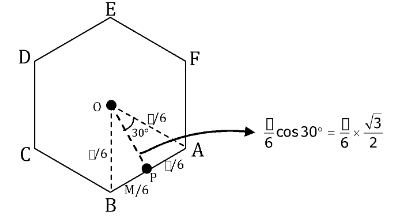MOI of AB about P : IABP = (M/6)(l/6)2/12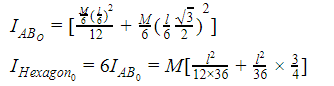= (6/100) [(24×24/12×36) + (24×24/36)×3/4]

= 0.8 kg m2

= 8×10-1 kg m2

Question 5: A point charge of +12 μC is at a distance 6 cm vertically above the centre of a square of side 12 cm as shown in figure. The magnitude of the electric flux through the square will be _____ ×103 Nm2/C.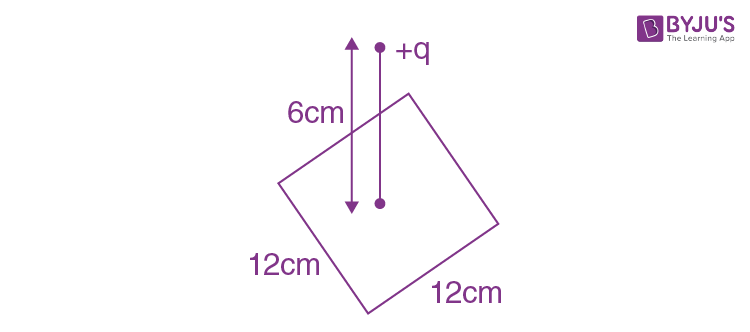Solution:

Using Gauss law, it is a part of cube of side 12 cm and charge at centre,

ϕ = Q/6ε0= 12μc/6ε0

$\frac{12\times 10^{-6}}{6\times 8.85\times10^{-12}}$

= 226×103 Nm2/C

Question 6: Two solids A and B of mass 1 kg and 2 kg respectively are moving with equal linear momentum. The ratio of their kinetic energies (K.E.)A : (K.E.)B will be A/1. So the value of A will be ________.

Solution:

Given that, M1/M2 = 1/2

we know that

K = p2/2M

K1/K2 = (p2/2M1 )×2M2/p2

K1/K2 = M2/M1 = 2/1

A/1 = 2/1

⇒∴ A = 2

Question 7: The root mean square speed of molecules of a given mass of a gas at 270C and 1 atmosphere pressure is 200 ms-1. The root mean square speed of molecules of the gas at 1270C and 2 atmosphere pressure is x/√3 ms-1. The value of x will be __________.

Solution:

Vrms = √(3RT1/M0)

200 = √(3R×300/M0) ....(1)

Also, x/√3 = √(3R×400/M0) …(2)

(1)÷(2)

200/(x/√3) = √(300/400) = √(3/4)

⇒x = 400 m/s

Question 8: A series LCR circuit is designed to resonate at an angular frequency ω0 = 105rad/s. The circuit draws 16W power from 120 V source at resonance. The value of resistance ‘R’ in the circuit is _______ Ω.

Solution:

P = V2/R

16 = 1202/R

⇒ R = 14400/16

⇒ R = 900 Ω

Question 9: An electromagnetic wave of frequency 3 GHz enters a dielectric medium of relative electric permittivity 2.25 from vacuum. The wavelength of this wave in that medium will be _______ ×10-2 cm.

Solution:

εr = 2.25

Assuming non-magnetic material ⇒ μr = 1

Hence refractive index of the medium

n = √(μrεr )= √2.25 = 1.5

∴λvm=n

λm = C/f.n

= 3×108/3×109×1.5

= (⅔) 10-1 m

= 667×10-2 cm

Question 10: A signal of 0.1 kW is transmitted in a cable. The attenuation of cable is -5 dB per km and cable length is 20 km. The power received at receiver is 10-xW. The value of x is ______.

[Gain in dB = 10 log10(P0/Pi)]

Solution:

Power of signal transmitted: Pi = 0.1 Kw = 100w

Rate of attenuation = -5 dB/Km

Total length of path = 20 km

Total loss suffered = -5×20 = -100dB

Gain in dB = 10 log10(P0/Pi)

-100 = 10log10(P0/Pi)

log10(Pi/P0) = 10

log10(Pi/P0) = log101010

100/P0 = 1010

⇒ P0 = 1/108 = 10-8

⇒ x = 8

### JEE Main 2021 Physics Paper February 24 Shift 2